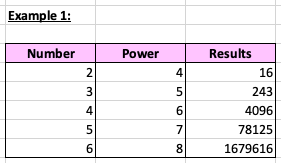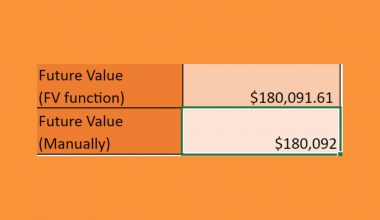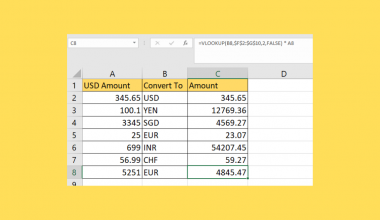# How to Do Exponential Calculations to a Range of Cells in Excel

Learning how to do exponential calculations to a range of cells in Microsoft Excel is useful to calculate repeated multiplications working with base and exponents.

Exponential numbers are a form of calculation by multiplying a number by itself and a number of times.

For example, 6x6x6=216 in exponential form is 63=216, where six is the base and three is the power. In this case, the power indicates the number of times the base is multiplied by itself.

You must be thinking, when will you ever use the exponential function other than when in math class. The application of the exponential function in real-life scenarios may surprise you as it is widely and often used.

For instance, we will use the exponential function when calculating the amount of money we will get with compound interest. Besides, researchers would also often apply exponential calculation when studying the increase of populations in a city.

Let’s learn more about exponential calculations in Excel by using different methods and formulas.

## Exponential Calculation to a Range of Cells Using the Power Function

Let’s learn to use the Power function to perform exponential calculations in Microsoft Excel. Before we dive into the application of the Power function, here is some information for your better understanding.

The Power function helps return results for a base number multiplied by itself for a stated amount of time.

The syntax of the `POWER` function is as follow:

`= POWER(number, power)`

Let us look at each term in the formula to understand what they mean:

• `=` is the equal sign which is how we start any function in Microsoft Excel.
• `POWER()` is our Power function. It returns the result after raising a base number to a power.
• `number` is a base number.
• `power` is the exponent that the base number is raised.

Now that we know the Power function, let’s use an example to demonstrate how to apply it in Excel.

You may make a copy of the spreadsheet using the link I have attached below.

#### Example 1:

1. First, let’s select the cell that will contain our Power function result. In this example, we will select D5 to insert the formula in.1. Next, we simply type the equal sign `=` to begin the function, followed by `POWER(`.1. You may find the Excel help box with a description of using the POWER function by clicking on the suggested text box. We can click on the red exit button located on the top-left corner of the box to minimize it.1. Next, we will type in our arguments. We will select B5 as the `number` and C5 as the `power`. Don’t forget to close the formula with a closing bracket `)`.1. Once we press Enter, the formula returns 16.1. Besides, you can also pull the tiny bottom-right corner downwards to the rest of the data. This helps to copy the same formula and apply it to the other cells.1. Once we press Enter, the rest of the cells are filled with returned results.## Exponential Calculation to a Range of Cells Using the Power Symbol

Other than using the Power function, we can use the power symbol in Excel to calculate exponential numbers.

The real name for the power symbol in Excel is called a caret ^. To visualize 52 is also 5^2.

Let’s use an example to see how to perform exponential calculations to a range of cells using the power symbol.

#### Example 2:

1. First, let’s select the cell that will contain our formula. In this example, we will select D5 to insert the formula in.1. Next, we simply type the equal sign `=` to begin the formula.1. Then, we select B5, insert the power symbol `^`, and select C5.1. Once we press Enter, the formula returns 16.1. Like the previous example, simply drag the bottom-right corner downwards to apply the formula to all remaining cells. Once we press Enter, the remaining results will appear!There you go! You can easily formulate exponential calculations in Microsoft Excel using the Power function or the power symbol. Exponential calculations can be applied to many more real-life scenarios and will come in handy!

Don’t forget to check out other cool functions in Microsoft Excel to enhance and simplify work for your everyday use!

Make sure to subscribe to our newsletter to be the first to know about the latest guides and tutorials from us.### Get emails from us about Microsoft Excel.

Our goal this year is to create lots of rich, bite-sized tutorials for Microsoft Excel users like you. If you liked this one, you'd love what we are working on! Readers receive ✨ early access ✨ to new content.

##### You May Also Like## How to Get Future Value of Annuity Formula in Excel

This guide will discuss how to get the future value of the annuity formula in Excel using the…## How to Generate a List of all Possible Permutations in Excel

This guide will explain how you can use Excel formulas to generate a list of all possible permutations…## How to Apply Different Types of Conditional Formatting in Excel

This guide will discuss how to apply different types of conditional formatting in Excel. Conditional formatting in Excel…## How to Make an Isometric Drawing in Excel

This guide will explain how to create an isometric drawing using Microsoft Excel. Isometric drawings are commonly used…